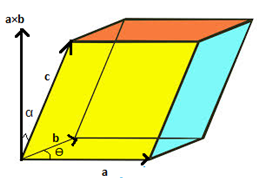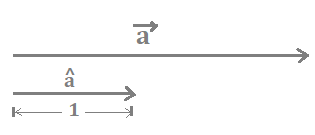# Vector and Its ApplicationsVectors are quantities with both magnitude and direction in contrast to scalars which only have magnitude. For example, speed and velocity, these two physical quantities, might seem similar, but there is a significant difference between them. Speed represents only the magnitude of the rate of change in motion of a body, whereas velocity represents both magnitude and direction. Some examples of physical vector quantities are displacement, acceleration, force, etc.

In mathematics, we treat vectors as fundamental elements of vector space on which we can perform various algebraic operations. Vector algebra constitutes the addition of vectors, subtraction of vectors, scalar multiplication, vector product, etc. Generally, vectors are represented as letters with an arrow on them. This article will represent vectors as bold letters for their distinction from scalar quantities.

A vector in a 2D Euclidean plane is written as a column of scalars.Where vx is the horizontal component of vector v, and vy is the vertical component.Magnitude of v = ||v|| = √(vx2 + vy2) and v = vxi + vyj, where i and j are unit vectors along the horizontal and vertical axis.

Vector addition and subtraction are quite similar to the addition and subtraction of algebraic expressions. We add or subtract like terms. Multiplication of vectors is of two types – scalar product, also known as the dot product, and the vector product or cross product.

In a scalar product, two vectors, A and B, are put into a binary operation, which results in a scalar quantity. That is,

A.B = |A| |B| cos ���; ��� is the angle between A and B.

It is also defined as the product of the magnitude of one vector with the projection of one vector on another.

In a vector product, two vectors, A and B, are put into a binary operation, resulting in a vector quantity. That is,

A × B = |A||B| sin ��� , where is the unit vector which is pointing out perpendicularly from the plane containing the vectors A and B. Since a cross sign denotes the vector product, thus it is called the cross product of the vectors. Therefore, the cross-product of two vectors lying in the same plane is the vector perpendicular to the plane containing both vectors.

Another type of vector multiplication is vector triple product – the product of three vectors in three-dimensional space. Vector triple product is also of two types – the scalar triple product or mixed product and vector triple product.

Suppose three vectors are there in a three-dimensional vector space such that,

A = a1î + a2ĵ + a3ƙ

B = b1î + b2ĵ + b3ƙ

C = c1î + c2ĵ + c3ƙ

The mixed product of these three vectors is defined as the scalar product of one vector with the cross product of the other two vectors.

 A . (B × C)

This triple product represents the volume of a parallelepiped whose length, breadth and height are the three vectors A, B and C, respectively.

The vector triple product of three vectors is defined as the vector product of one vector with the vector product of the other two.

 A × (B × C) = (A . C)B – (A . B)C

Another operation related to vectors is the scaling of vectors. Vectors can be scaled or translated by multiplying scalars to them. If a is a vector in a particular direction, then ���a is the same vector shifted by ��� distance in the same direction that of a. Therefore, a and ���a are parallel vectors, separated by a distance of ��� units. If ��� is a negative scalar, then a and –���a are anti-parallel vectors, vectors parallel to each other but opposite in direction.

Unit or direction vectors are types of vectors parallel to the given vector, but their length is unity. It is denoted letters with a cap over it.Thus, unit vector â = a/||a||.

There are many more operations and concepts related to the manipulation vectors. Vectors are extremely useful elements, especially in geometry and various physical quantities. The parallelogram law of the addition of vectors is crucial while explaining many phenomena of physics and engineering.